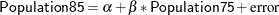Example 94.3 Regression Estimator for Simple Random Sample

By using auxiliary information, you can construct regression estimators to provide more accurate estimates of population characteristics. With ESTIMATE statements in PROC SURVEYREG, you can specify a regression estimator as a linear function of the regression parameters to estimate the population total. This example illustrates this application by using the data set Municipalities from Example 94.2.

In this sample, a linear model between the Swedish populations in 1975 and in 1985 is established:Assuming that the total population in 1975 is known to be 8200 (in thousands), you can use the ESTIMATE statement to predict the 1985 total population by using the following statements:

title1 'Regression Analysis for Swedish Municipalities';
title2 'Estimate Total Population';
proc surveyreg data=Municipalities total=50;
cluster Cluster;
model Population85=Population75;
estimate '1985 population' Intercept 284 Population75 8200;
run;

Since each observation in the sample is a municipality and there is a total of 284 municipalities in Sweden, the coefficient for Intercept () in the ESTIMATE statement is 284 and the coefficient for Population75 () is the total population in 1975 (8.2 million).

Output 94.3.1 displays the regression results and the estimation of the total population. By using the linear model, you can predict the total population in 1985 to be 8.64 million, with a standard error of 0.26 million.

Output 94.3.1: Use the Regression Estimator to Estimate the Population Total

 Regression Analysis for Swedish Municipalities Estimate Total Population

The SURVEYREG Procedure

Regression Analysis for Dependent Variable Population85

Estimate
Label Estimate Standard Error DF t Value Pr > |t|
1985 population 8642.49 258.56 4 33.43 <.0001